## NCERT solutions for class 6 mathematics -Exercise Solutions

### Chapter 05-Understanding Elementary Shapes-Exercise Solutions

NCERT Book Page Number-88
Q.1. What is the disadvantage in comparing line segments by mere observation?
Answer: By mere observation, we cannot be absolutely sure about the judgement. When we compare two line segments of almost same lengths, we cannot be sure about the line segment of greater length. Therefore, it is not an appropriate method to compare line segments having a slight difference between their lengths. This is the disadvantage in comparing line segments by mere observation.
Q.2. Why is it better to use a divider than a ruler, while measuring the length of a line segment?
Answer: It is better to use a divider than a ruler because while using a ruler, positioning error may occur due to the incorrect positioning of the eye.
Q.3. Draw any line segment, say $\overline AB$. Take any point C lying in between A and B. Measure the lengths of AB, BC and AC. Is AB = AC + CB?
[Note: If A, B, C are any three points on a line such that AC + CB = AB, then we can be sure that C lies between A and B]
Answer:
It is given that point C is lying somewhere in between A and B. Therefore, all these points are lying on the same line segment$\overline AB$. Hence, for every situation in which point C is lying in between A and B, it may be said that AB = AC + CB.
For example,
$\overline AB$ is a line segment of 6 cm and C is a point between A and B, such that it is 2 cm away from point B. We can find that the measure of line segment $\overline AC$ comes to 4 cm.
Hence, relation AB = AC + CB is verified.
NCERT Book Page Number-89

Q.4. If A, B, C are three points on a line such that AB = 5 cm, BC = 3 cm and AC = 8 cm, which one of them lies between the other two?
Answer: Given that,
AB = 5 cm
BC = 3 cm
AC = 8 cm
It can be observed that AC = AB + BC
Clearly, point B is lying between A and C.

Q.5. Verify, whether D is the mid point of $\overline AG$ .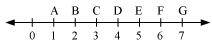Answer:
From the given figure, it can be observed that
$\overline AD$= 4 − 1 = 3 units
$\overline DG$= 7 − 4 = 3 units
$\overline AG$ = 7 − 1 = 6 units
Clearly, D is the mid-point of AG.
Q.6. If B is the mid point of $\overline AC$ and C is the mid point of$\overline BD$, where A, B, C, D lie on a straight line, say why AB = CD?
Answer: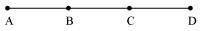Since B is the mid-point of AC,
AB = BC (1)
Since C is the mid-point of BD,
BC = CD (2)
From equation (1) and (2), we may find that
AB = CD
NCERT Book Page Number-91
Q.1. What fraction of a clock wise revolution does the hour hand of a clock turn through when it goes from
(a) 3 to 9
(b) 4 to 7
(c) 7 to 10
(d) 12 to 9
(e) 1 to 10
(f) 6 to 3
Answer: We may observe that in 1 complete clockwise revolution, the hour hand will rotate by 360º.
(a) When the hour hand goes from 3 to 9 clockwise, it will rotate by 2 right angles or 180º.
Fraction = $\frac{{180}^{o}}{{360}^{o}}$
$=\frac{1}{2}$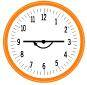(b) When the hour hand goes from 4 to 7 clockwise, it will rotate by 1 right angle or 90º.
Fraction = $\frac{{90}^{o}}{{360}^{o}}$
$=\frac{1}{4}$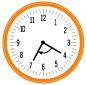(c) When the hour hand goes from 7 to 10 clockwise, it will rotate by 1 right angle or 90º.
Fraction = $\frac{{90}^{o}}{{360}^{o}}$
$=\frac{1}{4}$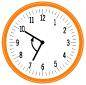(d) When the hour hand goes from 12 to 9 clockwise, it will rotate by 3 right angles or 270º.
Fraction = $\frac{{270}^{o}}{{360}^{o}}$
$=\frac{3}{4}$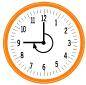(e) When the hour hand goes from 1 to 10 clockwise, it will rotate by 3 right angles or $270^{0}$.
Fraction = $\frac{{270}^{o}}{{360}^{o}}$
$=\frac{3}{4}$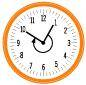(f) When the hour hand goes from 6 to 3 clockwise, it will rotate by 3 right angles or $270^{0}$.
Fraction = $\frac{{270}^{o}}{{360}^{o}}$
$=\frac{3}{4}$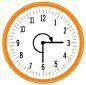Q.2. Where will the hand of a clock stop if it
(a) Starts at 12 and makes $\frac{1}{2}$ of a revolution, clockwise?
(b) Starts at 2 and makes$\frac{1}{2}$ of a revolution, clockwise?
(c) Starts at 5 and makes $\frac{1}{4}$ of a revolution, clockwise?
(d) Starts at 5 and makes $\frac{3}{4}$ of a revolution, clockwise?
Answer:
In 1 complete clockwise revolution, the hand of a clock will rotate by $360^{0}$.
(a) If the hand of the clock starts at 12 and makes $\frac{1}{2}$ of a revolution clockwise, then it will rotate by 180º and hence, it will stop at 6.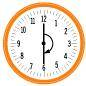(b) If the hand of the clock starts at 2 and makes $\frac{1}{2}$ of a revolution clockwise, then it will rotate by 180º and hence, it will stop at 8.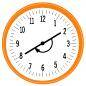(c) If the hand of the clock starts at 5 and makes $\frac{1}{4}$ of a revolution clockwise, then it will rotate by $90^{0}$ and hence, it will stop at 8.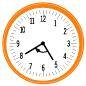(d) If the hand of the clock starts at 5 and makes $\frac{3}{4}$ of a revolution clockwise, then it will rotate by $270^{0}$ and hence, it will stop at 2.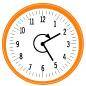Q.3. Which direction will you face if you start facing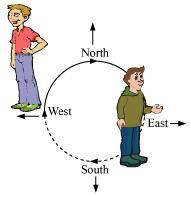(a) East and make $\frac{1}{2}$ of a revolution clockwise?
(b) East and make1$\frac{1}{2}$ of a revolution clockwise?
(c) West and make $\frac{3}{4}$ of a revolution anti-clockwise?
(d) South and make one full revolution?
(Should we specify clockwise or anti-clockwise for this last question? Why not? )
Answer:
If we revolve one complete round in either clockwise or anti-clockwise direction, then we will revolve by $360^{0}$ and the two adjacent directions will be at $90^{0}$ or $\frac{1}{4}$ of a complete revolution away from each other.
(a) If we start facing East and make $\frac{1}{2}$ of a revolution clockwise, then we will face the West direction.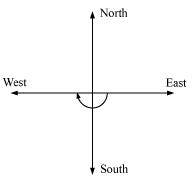(b) If we start facing East and make 1$\frac{1}{2}$ of a revolution clockwise, then we will face the West direction.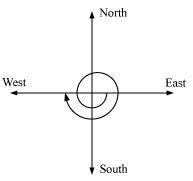(c) If we start facing West and make $\frac{3}{4}$ of a revolution anti-clockwise, then we will face the North direction.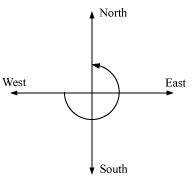(d) If we start facing South and make a full revolution, then we will again
face the South direction.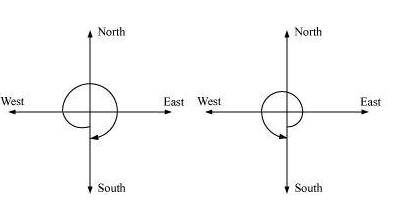In case of revolving by 1 complete round, the direction in which we are revolving does not matter. In both cases, clockwise or anti-clockwise, we will be back at our initial position.

Q.4. What part of a revolution have you turned through if you stand facing
(a) East and turn clock wise to face north?
(b) South and turn clockwise to face east?
(c) West and turn clockwise to face east?
Answer:
If we revolve one complete round in either clockwise or anti-clockwise direction, then we will revolve by 360º and the two adjacent directions will be at 90º or $\frac{1}{4}$ of a complete revolution away from each other.
(a) If we start facing East and turn clockwise to face North, then we have to make $\frac{3}{4}$ of a revolution.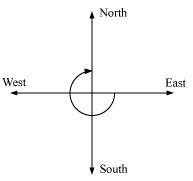(b) If we start facing South and turn clockwise to face east, then we have to make$\frac{3}{4}$ of a revolution.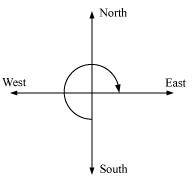(c) If we start facing West and turn clockwise to face East, then we have to make $\frac{1}{2}$ of a revolution.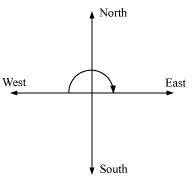Q.5. Find the number of right angles turned through by the hour hand of a clock when it goes from
(a) 3 to 6 (b) 2 to 8 (c) 5 to 11
(d) 10 to 1 (e) 12 to 9 (f) 12 to 6
Answer:
The hour hand of a clock revolves by $360^{0}$ or 4 right angles in 1 complete round.
(a) The hour hand of a clock revolves by $90^{0}$ or 1 right angle when it goes from 3 to 6.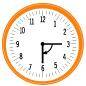(b) The hour hand of a clock revolves by $180^{0}$ or 2 right angles when it goes from 2 to 8.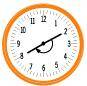(c) The hour hand of a clock revolves by $180^{0}$ or 2 right angles when it goes from 5 to 11.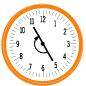(d) The hour hand of a clock revolves by $90^{0}$ or 1 right angle when it goes from 10 to 1.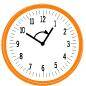(e) The hour hand of a clock revolves by $270^{0}$ or 3 right angles when it goes from 12 to 9.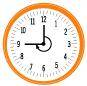(f) The hour hand of a clock revolves by $180^{0}$ or 2 right angles when it goes from 12 to 6.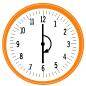NCERT Book Page Number-92

Q.6. How many right angles do you make if you start facing
(a) South and turn clockwise to west?
(b) North and turn anti-clockwise to east?
(c) West and turn to west?
(d) South and turn to north?
Answer:
If we revolve one complete round in either clockwise or anti-clockwise direction, then we will revolve by 360º or 4 right angles and the two adjacent directions will be at 90º or 1 right angle away from each other.
(a) If we start facing South and turn clockwise to West, then we make 1 right angle.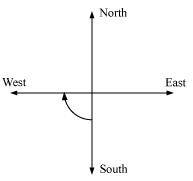(b) If we start facing North and turn anti-clockwise to East, then we make 3 right angles.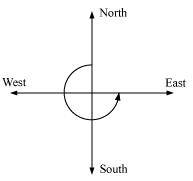(c) If we start facing West and turn to West, then we make 1 complete round or 4 right angles.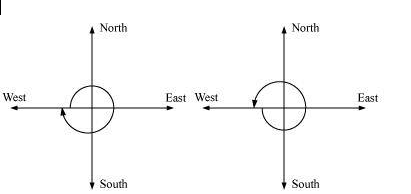(d) If we start facing South and turn to North, then we make 2 right angles.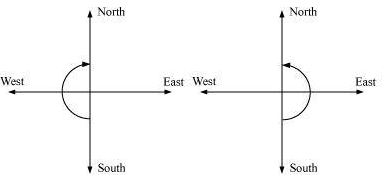Q.7. Where will the hour hand of a clock stop if it starts
(a) From 6 and turns through 1 right angle?
(b) From 8 and turns through 2 right angles?
(c) From 10 and turns through 3 right angles?
(d) From 7 and turns through 2 straight angles?
Answer:
In 1 complete revolution (clockwise or anti-clockwise), the hour hand of a clock will rotate by 360º or 4 right angles.
1. If the hour hand of a clock starts from 6 and turns through 1 right angle, then it will stop at 9.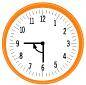2. If the hour hand of a clock starts from 8 and turns through 2 right angles, then it will stop at 2.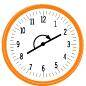3. If the hour hand of a clock starts from 10 and turns through 3 right angles, then it will stop at 7.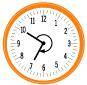4. If the hour hand of a clock starts from 7 and turns through 2 straight angles, then it will stop at 7.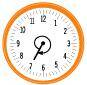NCERT Book Page Number-94
Q.1. Match the following: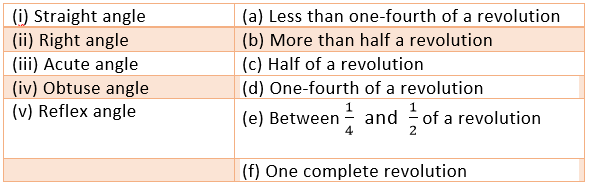Answer:
(i) Straight angle is of 180º and half of a revolution is 180º.
Hence, (i) ↔ (c)
(ii) Right angle is of 90º and one-fourth of a revolution is 90º.
Hence, (ii) ↔ (d)
(iii) Acute angles are the angles less than 90º. Also, less than one-fourth of a revolution is the angle less than 90º.
Hence, (iii) ↔ (a)
(iv) Obtuse angles are the angles greater than 90º but less than 180º. Also, between$\frac{1}{4}$ and $\frac{1}{2}$ of a revolution is the angle whose measure lies between 90º and 180º.
Hence, (iv) ↔ (e)
(v) Reflex angles are the angles greater than 180º but less than 360º. Also, more than half a revolution is the angle whose measure is greater than 180º.
Hence, (v) ↔ (b)
NCERT Book Page Number-95
Q.1. Classify each one of the following angles as right, straight, acute, obtuse or reflex: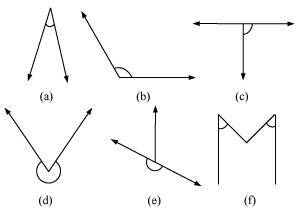Answer:
(a) Acute angle as its measure is less than 90º.
(b) Obtuse angle as its measure is more than 90º but less than 180º.
(c) Right angle as its measure is 90º.
(d) Reflex angle as its measure is more than 180º but less than 360º.
(e) Straight angle as its measure is 180º.
(f) Acute angle as its measure is less than 90º.
NCERT Book Page Number-97

Q.1. What is the measure of (i) a right angle? (ii) a straight angle?
Answer:
(i) The measure of a right angle is 90°.
(ii) The measure of a straight angle is 180°.
Q.2. Say True or False:
(a) The measure of an acute angle < 90°
(b) The measure of an obtuse angle < 90° (c) The measure of a reflex angle > 180°
(d) The measure of one complete revolution = 360°
(e) If m∠A = 53° and m∠B = 35°, then m∠A > m∠B.
Answer:
(a) True
The measure of an acute angle is less than 90°.
(b) False
The measure of an obtuse angle is greater than 90º but less than 180º.
(c) True
The measure of a reflex angle is greater than 180°.
(d) True
The measure of one complete revolution is 360º.
(e) True
Q.3. Write down the measures of
(a) Some acute angles. (b) Some obtuse angles.
(Give at least two examples of each).
Answer:
(a) 45°, 70°
(b) 105°, 132°
Q.4. Measure the angles given below using the Protractor and write down the measure.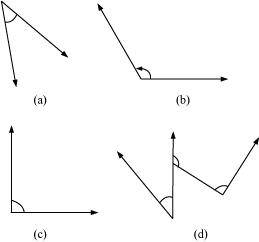Answer:
(a) 45º
(b) 120º
(c) 90º
(d) 60º, 90º, and 130º

NCERT Book Page Number-98
Q.5. Which angle has a large measure? First estimate and then measure.
Measure of angle A =
Measure of angle B =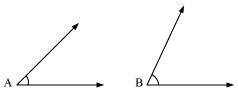Answer:
Measure of angle A = 40º
Measure of angle B = 68º
∠B has the greater measure than ∠A.
Q.6. From these two angles which has larger measure? Estimate and then confirm by measuring them.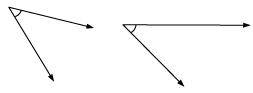Answer:
The measures of these angles are 45º and 55º. Therefore, the angle shown in 2nd figure is greater.
Q.7. Fill in the blanks with acute, obtuse, right or straight:
(a) An angle whose measure is less than that of a right angle is _______.
(b) An angle whose measure is greater than that of a right angle is _______.
(c) An angle whose measure is the sum of the measures of two right angles is _______.
(d) When the sum of the measures of two angles is that of a right angle, then each one of them is _______.
(e) When the sum of the measures of two angles is that of a straight angle, and if one of them is acute then the other should be _______.
Answer:
(a) Acute angle
(b) Obtuse angle (if the angle is less than 180º)
(c) Straight angle
(d) Acute angle
(e) Obtuse angle
Q.8. Find the measure of the angle shown in each figure. (First estimate with your eyes and then find the actual measure with a protractor).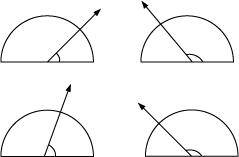Answer: The measures of the angles shown in the above figure are 40º, 130º, 65º, 135º respectively.
Q.9. Find the angle measure between the hands of the clock in each figure: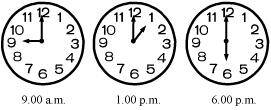Answer:
(a) 90°
(b) 30°
(c) 180°
NCERT Book Page Number-99
Q.10. Investigate
In the given figure, the angle measures 30°. Look at the same figure through a magnifying glass. Does the angle become larger? Does the size of the angle change?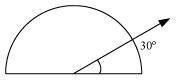Answer:
The measure of this angle will not change.
Q.11. Measure and classify each angle: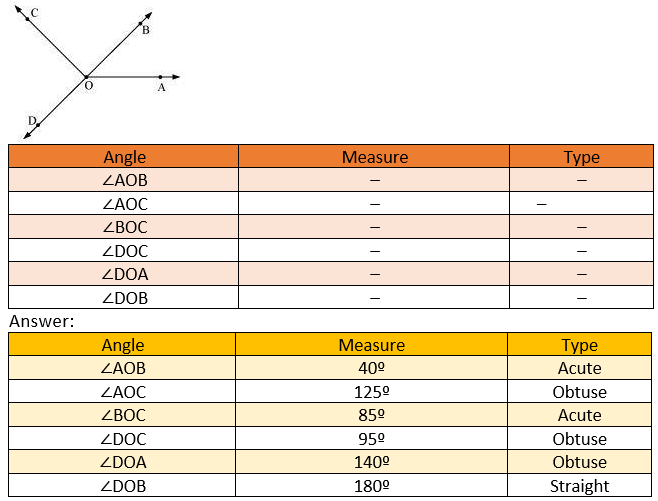NCERT Book Page Number-100
Q.1. Which of the following are models for perpendicular lines:
(a) The adjacent edges of a table top.
(b) The lines of a railway track.
(c) The line segments forming the letter ’L’
(d) The letter V.
Answer:
(a) The adjacent edges of a table top are perpendicular to each other.
(b) The lines of a railway track are parallel to each other.
(c) The line segments forming the letter ’L’ are perpendicular to each other.
(d) The sides of letter V are inclined at some acute angle on each other.
Hence, (a) and (c) are the models for perpendicular lines.
Question 2:
Q.2. Let $\overline{PQ}$ be the perpendicular to the line segment $\overline{XY}$. Let $\overline{PQ}$ and $\overline{PQ}$ intersect in the point A. What is the measure of ∠PAY?
Answer: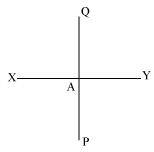From the figure, it can be easily observed that the measure of ∠PAY is 90°.

Q.3. There are two set-squares in your box. What are the measures of the angles that are formed at their corners? Do they have any angle measure that is common?
Answer: One has a measure of 90°, 45°, 45°.
Other has a measure of 90°, 30°, 60°.
Therefore, the angle of 90° measure is common between them.

Q.4. Study the diagram. The line l is perpendicular to line m.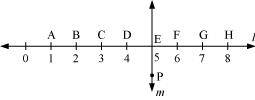(a) Is CE = EG?
(b) Does PE bisect CG?
(c) Identify any two line segments for which PE is the perpendicular bisector.
(d) Are these true?
(i) AC > FG.
(ii) CD = GH.
(iii) BC < EH.
Answer:
(a) Yes. As CE = EG = 2 units
(b) Yes. PE bisects CG since CE = EG.
(c) and
(d) (i) True. As length of AC and FG are of 2 units and 1 unit respectively.
(ii) True. As both have 1 unit length.
(iii) True. As the length of BC and EH are of 1 unit and 3 units respectively.
NCERT Book Page Number-103
Q.1. Name the types of following triangles:
(a) Triangle with lengths of sides 7 cm, 8 cm and 9 cm.
(b) ΔABC with AB = 8.7 cm, AC = 7 cm and BC = 6 cm.
(c) ΔPQR such that PQ = QR = PR = 5 cm.
(d) ΔDEF with m∠D = 90°
(e) ΔXYZ with m∠Y = 90° and XY = YZ.
(f) ΔLMN with m∠L = 30°, m∠M = 70° and m∠N = 80°
Answer:
(a) Scalene triangle
(b) Scalene triangle
(c) Equilateral triangle
(d) Right-angled triangle
(e) Right-angled isosceles triangle
(f) Acute-angled triangle
Q.2. Match the following: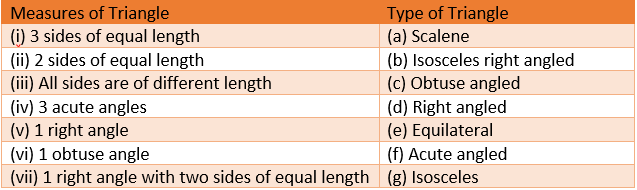Answer:
(i) Equilateral (e)
(ii) Isosceles (g)
(iii) Scalene (a)
(iv) Acute-angled (f)
(v) Right-angled (d)
(vi) Obtuse-angled (c)
(vii) Isosceles right-angled (b)
Q.3. Name each of the following triangles in two different ways: (you may judge the nature of the angle by observation)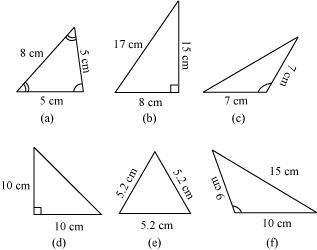Answer:
(a) Acute-angled and isosceles
(b) Right-angled and scalene
(c) Obtuse-angled and isosceles
(d) Right-angled and isosceles
(e) Acute-angled and equilateral
(f) Obtuse-angled and scalene
NCERT Book Page Number-104
Q.4. Try to construct triangles using match sticks. Some are shown here. Can you make a triangle with
(a) 3 matchsticks?
(b) 4 matchsticks?
(c) 5 matchsticks?
(d)6 matchsticks?
(Remember you have to use all the available matchsticks in each case)
Name the type of triangle in each case. If you cannot make a triangle, think of reasons for it.
Answer:
(a) By using 3 matchsticks, we can form a triangle as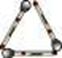(b) By using 4 matchsticks, we cannot form a triangle. This is because the sum of the lengths of any two sides of a triangle is always greater than the length of the remaining side of the triangle.
(c) By using 5 matchsticks, we can form a triangle as